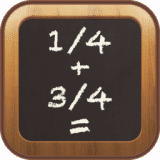Fractions-Calculator
1.1

0.0

Fractions-Calculator# Fractions-Calculator1.1

04 May 2015

Easy way to work with fractions.

## Overview

Fractions-Calculator is the easiest way to work with fractions. It’s a great help to validate your own calculations and solve fraction problems. Fractions-Calculator helps you add, subtract, multiply, divide, compare, and convert fractions, improper fractions, and mixed numbers. The app includes the following fraction problems:

• Simple Fractions
• Mixed Fractions
• Decimal-to-Fractions
• Fraction-to-Decimal
• Fraction-to-Percent
• Percent-to-Fraction
• Mixed Number-to-Decimal
• Mixed Number-to-Percent
• Mixed Number-to-Improper Fractions
• Comparing Fractions

Fractions-Calculator is a great app for anyone who needs to learn these concepts. The calculator will generate a detailed step-by-step explanation for each operation.

## What's new in Fractions-Calculator

Version 1.1:
• Initial public release

#### Join over 500,000 subscribers.

Subscribe for our newsletter with best Mac offers from MacUpdate.

How would you rate Fractions-Calculator app?